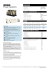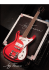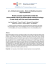# slides

## Transcription

slides
```Math Modeling Acoustic Model Simulations Conclusion
Finding Buried Targets Using Acoustic
Excitation
Zackary R. Kenz
In collaboration with Dr. Shuhua Hu, Dr. Grace Kepler, Clay Thompson – NCSU,
L3 Communications team led by Dr. Jerrold Levine,
and Dr. Richard Albanese
Center for Research in Scientific Computation
Department of Mathematics
North Carolina State University
October 7, 2010
Z.R. Kenz
Acoustic/EM Target Detection
Math Modeling Acoustic Model Simulations Conclusion
1
Math Modeling and Differential Equations
2
1D Model Formulation
3
Simulation Setup and Results
4
Conclusion
Z.R. Kenz
Acoustic/EM Target Detection
Math Modeling Acoustic Model Simulations Conclusion
Goals for Math Modeling Portion
Introduction to math modeling
Introduce differential equations
Examine how changing parts of a differential equation can
change resulting behavior
Z.R. Kenz
Acoustic/EM Target Detection
Math Modeling Acoustic Model Simulations Conclusion
Goals for Target Detection Portion
Acoustic Dynamics
Given an impact to the soil, what sort of wave propagation
dynamics are expected?
How do changes in soil properties affect dynamics?
Electromagnetic Signal Dynamics
Given an arbitrarily moving target in the soil, what will a
Device Development
Z.R. Kenz
Acoustic/EM Target Detection
Math Modeling Acoustic Model Simulations Conclusion
1
Math Modeling and Differential Equations
2
1D Model Formulation
3
Simulation Setup and Results
4
Conclusion
Z.R. Kenz
Acoustic/EM Target Detection
Math Modeling Acoustic Model Simulations Conclusion
Math Modeling Overview
Modeling is developing equations to explain some
phenomenon, and then using the equations to make
Validating the equations with real life data is of particular
interest.
Z.R. Kenz
Acoustic/EM Target Detection
Math Modeling Acoustic Model Simulations Conclusion
Modeling Cycle
The Iterative Modeling Process
(iii) Abstraction or Mathematization
resulting in a mathematical model
(ii)
Formalization of properties,
relationships and mechanisms
which result in a biological or
physical model
(iv)
(i) Empirical Observations
(v)
(experiments and data collection)
(vii)
Changes in understanding of
mechanisms, etc., in the real
system.
Formation Stage: (i),(ii),(iii),(iv)
Formalization of
Uncertainty/Variablity in model and
data resulting in a statistical model
(vi)
Interpretation and Comparison
(with the real system)
Solution Stage: (v)
Z.R. Kenz
Model Analysis
Interpretation Stage: (vi), (vii)
Acoustic/EM Target Detection
Math Modeling Acoustic Model Simulations Conclusion
Differential Equations and Modeling
Modeling often uses differential equations to describe
relationships between variables
There are "ordinary" and "partial" differential equations
Question: What are differential equations?
Z.R. Kenz
Acoustic/EM Target Detection
Math Modeling Acoustic Model Simulations Conclusion
What is an ODE?
A differential equation is a mathematical equation for an
unknown function of one or several variables that relates the
values of the function itself and its derivatives of various orders.
An ordinary differential equation means the function of interest
depends only on one independent variable.
Instead of solving for numbers, like solving for x in x 2 − 1 = 0,
we are solving for a function
Z.R. Kenz
Acoustic/EM Target Detection
Math Modeling Acoustic Model Simulations Conclusion
ODE Example 1
dy(t)
=2
dt
The left hand side is the rate of change of the solution y(t).
The equation says that we want the rate of change of the
unknown function y(t) to be a constant.
What simple function has a constant rate of change (slope)?
Z.R. Kenz
Acoustic/EM Target Detection
Math Modeling Acoustic Model Simulations Conclusion
Example 1 Solution
dy(t)
=2
dt
If we integrate both sides with respect to t, the solution is
y(t) = 2t + c
c is a "constant of integration"
This could describe the motion of someone walking down the
sidewalk at a constant rate.
Z.R. Kenz
Acoustic/EM Target Detection
Math Modeling Acoustic Model Simulations Conclusion
Solution from previous slide: y(t) = 2t + c
In a particular problem, we might want the person to start
walking at position 5. Mathematically, we write y(0) = 5.
Applying that condition to the problem:
y(0) = 2 · 0 + c = 5.
Solving gives c = 5.
The full solution would then be
y(t) = 2t + 5
Z.R. Kenz
Acoustic/EM Target Detection
Math Modeling Acoustic Model Simulations Conclusion
Components of an ODE Problem
A full ODE problem is both the main equation (which includes
the derivatives) and also some conditions like our y(0) = 5.
The number of conditions depends on the highest number of
derivatives you have.
Now we’ll look at a slightly more complicated example.
Z.R. Kenz
Acoustic/EM Target Detection
Math Modeling Acoustic Model Simulations Conclusion
ODE Example 2
dy(t)
= 2y(t)
dt
This equation says that the rate of change of the solution is
proportional to the value of the function at every point t.
It’s a little more complicated to get the solution for this case, so
we won’t solve it here but just state the answer:
y(t) = e2t + c.
Changing the right hand side of the ODE from a constant to the
unknown function changed the solution behavior.
Z.R. Kenz
Acoustic/EM Target Detection
Math Modeling Acoustic Model Simulations Conclusion
ODE Example 3
dz(t)
= −2z(t)
dt
By changing the constant to −2, the solution is now
z(t) = e−2t + d.
Z.R. Kenz
Acoustic/EM Target Detection
Math Modeling Acoustic Model Simulations Conclusion
Example 2 vs Example 3
We’ll set the constants of integration equal to 0 (arbitrary).
We can compare the two solutions y(t) = e2t and z(t) = e−2t in
the figure below.
y(t)
z(t)
Function Value
6
4
2
0
0.1
0.2
0.3
0.4
Z.R. Kenz
0.5
t
0.6
0.7
0.8
Acoustic/EM Target Detection
0.9
1
Math Modeling Acoustic Model Simulations Conclusion
A Little More on ODEs
These equations can get more complicated. For example,
Higher derivatives can be in the equation
Parameters can be in the model.
The spring system is a common example:
m
d 2 y(t)
dy(t)
+c
+ k y(t) = 0
dt
dt 2
m: mass
c: damping
Z.R. Kenz
k: stiffness
Acoustic/EM Target Detection
Math Modeling Acoustic Model Simulations Conclusion
Example Solution for Spring System
Damped Spring System
2
Postion x(t)
1.5
1
0.5
0
−0.5
0
2
4
6
8
10
Time t
Z.R. Kenz
Acoustic/EM Target Detection
Math Modeling Acoustic Model Simulations Conclusion
Brief PDE Discussion
What if we want the solution to depend on multiple independent
variables, like the three spatial dimensions?
A partial differential equation (PDE) seeks a solution with more
than one independent variable. For example, we might want to
track the temperature of a room over time. We would then be
trying to find a function like
T (x, y, z, t).
Z.R. Kenz
Acoustic/EM Target Detection
Math Modeling Acoustic Model Simulations Conclusion
Brief PDE Discussion
Derivatives are now "partial" derivatives (denoted with ∂),
meaning with respect to one variable holding the others
constant.
For example, if we wanted the rate of change of temperature
with respect to time, we’d denote that quantity as
∂T (x, y, z, t)
.
∂t
Z.R. Kenz
Acoustic/EM Target Detection
Math Modeling Acoustic Model Simulations Conclusion
The Wave Equation
An equation that can be used to describe simple wave
motion in one spatial dimension is
2
∂ 2 u(z, t)
2 ∂ u(z, t)
=
.
c
∂t 2
∂z 2
Upon solving analytically, the constant c represents the
speed of the wave.
This particular form of the wave equation can be solved by
hand, but more complicated forms are solved numerically.
There is a shorthand notation for partial derivatives that turns the
wave equation above into utt = c 2 uzz .
Z.R. Kenz
Acoustic/EM Target Detection
Math Modeling Acoustic Model Simulations Conclusion
Moving Forward
With this brief introduction to modeling and differential
equations, we can start discussing my research project of
detecting buried targets.
The project is an example of the modeling cycle.
Z.R. Kenz
Acoustic/EM Target Detection
Math Modeling Acoustic Model Simulations Conclusion
1
Math Modeling and Differential Equations
2
1D Model Formulation
3
Simulation Setup and Results
4
Conclusion
Z.R. Kenz
Acoustic/EM Target Detection
Math Modeling Acoustic Model Simulations Conclusion
Definitions
Elastic material: returns to its original shape after applying
stress forces (rubber band)
Viscous material: Resists stresses (tar)
Viscoelastic material: Has both properties (rubber)
Z.R. Kenz
Acoustic/EM Target Detection
Math Modeling Acoustic Model Simulations Conclusion
Problem Situation
Open field where we want to detect buried objects
Thump in one area, study wave propagation outward from
thumper
Likely need a 2D or 3D model to capture full dynamics
As a first approximation, we’ll use a 1D model see which
features of wave propagation we can capture
Z.R. Kenz
Acoustic/EM Target Detection
Math Modeling Acoustic Model Simulations Conclusion
1D Problem
One dimensional problem schematic:
.
air
soil
.
soil
Observations of the wave form at a particular depth will be
taken at the z10 position
Z.R. Kenz
Acoustic/EM Target Detection
Math Modeling Acoustic Model Simulations Conclusion
1D Model: Key Assumptions
Both soil and target are uniform in horizontal (i.e., x and y)
directions
The column is a continuum
Dry soil behaves as a Kelvin-Voigt viscoelastic solid for
small-amplitude vibrations1
1
B.O. Hardin, The nature of damping in sands, J. Soil Mech. Found. Div.,
91 (1965), 63-97.
Z.R. Kenz
Acoustic/EM Target Detection
Math Modeling Acoustic Model Simulations Conclusion
1D Model: Notation
u(z, t) denotes the displacement (units: m) in the
z-direction at position z at time t
2
u(z,t)
Kelvin-Voigt stress: σ = κ ∂u(z,t)
+ η ∂ ∂t∂z
∂z
κ: elastic modulus (i.e. stiffness) in
η: damping coefficient in
ρ: soil density in
kg
m·s2
= Pa
kg
m·s
kg
m3
Z.R. Kenz
Acoustic/EM Target Detection
Math Modeling Acoustic Model Simulations Conclusion
1D Model: Only Soil
From the equations of motion for a continuum, we obtain
ρ∂
2 u(z,t)
∂t 2
=
=
∂
∂z
∂
∂z
(σ)
∂ 2 u(z,t)
κ ∂u(z,t)
+
η
∂z
∂t∂z
Remember from the wave equation slide that the wave speed was the
constant c in utt = c 2 uzz . For our model above, if we neglect damping
p
we get c = κ/ρ.
Z.R. Kenz
Acoustic/EM Target Detection
Math Modeling Acoustic Model Simulations Conclusion
1D Model: Including Rigid Target
Model the rigid target as a point mass in the column at z10 :
2
∂u(z,t)
∂ 2 u(z,t)
∂
κ
, z ∈ (zp0 , z10 ) ∪ (z10 , ∞)
ρ ∂ u(z,t)
=
+
η
∂z
∂z
∂t∂z
∂t 2
M∂
2 u(z ,t)
10
∂t 2
+
,t)
∂ 2 u(z10
∂u(z + ,t)
= S κ ∂z10 + η ∂t∂z
−
∂u(z − ,t)
∂ 2 u(z10
,t)
−S κ ∂z10 + η ∂t∂z
.
M: mass in kg of target
S: surface area in m2 of contact between target and soil under
(over) target
Z.R. Kenz
Acoustic/EM Target Detection
Math Modeling Acoustic Model Simulations Conclusion
1D Model: Additional Assumptions and Conditions
Assume zero displacement and zero velocity initially:
∂
u(z, 0) = 0
∂z
u(z, 0) = 0
At the surface, the normal internal stress is balanced with
the applied input force:
∂ 2 u(z,t) κ ∂u(z,t)
+
η
= −f (t),
∂z
∂t∂z
z=zp0
Computational assumption: the column is finite:
u(z00 , t) = 0
Z.R. Kenz
Acoustic/EM Target Detection
Math Modeling Acoustic Model Simulations Conclusion
1
Math Modeling and Differential Equations
2
1D Model Formulation
3
Simulation Setup and Results
4
Conclusion
Z.R. Kenz
Acoustic/EM Target Detection
Math Modeling Acoustic Model Simulations Conclusion
Simulation Setup
Surface: zp0 = 0m
Observation point/target: z10 = 0.3048m
Far boundary: z00 = 50m
Z.R. Kenz
Acoustic/EM Target Detection
Math Modeling Acoustic Model Simulations Conclusion
Simulation Setup
Forcing Function
5
4
x 10
3
f(t) (units:N/m2)
2
1
0
−1
−2
−3
−4
0
0.002
0.004
0.006
t (units: s)
Z.R. Kenz
0.008
0.01
Acoustic/EM Target Detection
Math Modeling Acoustic Model Simulations Conclusion
If soil density ρ changes, what happens to the wave form
and speed?
What happens if the elastic modulus κ changes?
What happens when the wave impacts the rigid body
target?
This is the Model Analysis part of the modeling cycle.
Z.R. Kenz
Acoustic/EM Target Detection
Math Modeling Acoustic Model Simulations Conclusion
Results, Soil Only
Changes in Soil: Density
−4
10
x 10
Displacement around z=0.3048m (z=1 ft)
κ=204000000 ρ=1440
κ=204000000 ρ=1800
κ=204000000 ρ=2250
u(0.3048,t) (units: m)
8
6
4
2
0
−2
0
0.002
0.004
0.006
0.008
0.01
t (units: s)
Wave speed heuristic (no damping): v ≈
Z.R. Kenz
p
κ/ρ
Acoustic/EM Target Detection
Math Modeling Acoustic Model Simulations Conclusion
Results, Soil Only
Changes in Soil: Elastic Modulus
−4
12
x 10
Displacement around z=0.3048m (z=1 ft)
κ=102000000 ρ=1800
κ=204000000 ρ=1800
κ=408000000 ρ=1800
u(0.3048,t) (units: m)
10
8
6
4
2
0
−2
0
0.002
0.004
0.006
0.008
0.01
t (units: s)
Heuristic: v ≈
p
κ/ρ
Z.R. Kenz
Acoustic/EM Target Detection
Math Modeling Acoustic Model Simulations Conclusion
Results, Soil Only
Changes in Soil: Density and Elastic Modulus
−4
14
x 10
Displacement around z=0.3048m (z=1 ft)
κ=102000000 ρ=1440
κ=204000000 ρ=1800
κ=408000000 ρ=2250
u(0.3048,t) (units: m)
12
10
8
6
4
2
0
−2
0
0.002
0.004
0.006
0.008
0.01
t (units: s)
Heuristic: v ≈
p
κ/ρ
Z.R. Kenz
Acoustic/EM Target Detection
Math Modeling Acoustic Model Simulations Conclusion
Results, Soil Only
−4
12
x 10
Displacement around z=0.3048m (z=1 ft)
κ=163200000 ρ=1440
κ=204000000 ρ=1800
κ=255000000 ρ=2250
u(0.3048,t) (units: m)
10
8
6
4
2
0
−2
0
0.002
0.004
0.006
0.008
0.01
t (units: s)
Heuristic: v ≈
p
κ/ρ
Z.R. Kenz
Acoustic/EM Target Detection
Math Modeling Acoustic Model Simulations Conclusion
Results, With Target: Wave Form Passing by Target
Wave form in z−domain, at time t=0.00067831
−5
14
x 10
Displacement, units: m
12
10
8
6
4
2
0
−2
0
0.5
1
1.5
2
Distance under ground, units: m
Dashed line represents location of target in column
Z.R. Kenz
Acoustic/EM Target Detection
Math Modeling Acoustic Model Simulations Conclusion
Results, With Target: Wave Form Passing by Target
Wave
form in z−domain, at time t=0.0020486
−4
x 10
Displacement, units: m
8
6
4
2
0
0
0.5
1
1.5
2
Distance under ground, units: m
Z.R. Kenz
Acoustic/EM Target Detection
Math Modeling Acoustic Model Simulations Conclusion
Results, With Target: Wave Form Passing by Target
Wave
form in z−domain, at time t=0.0054744
−4
x 10
Displacement, units: m
8
6
4
2
0
0
0.5
1
1.5
2
Distance under ground, units: m
Z.R. Kenz
Acoustic/EM Target Detection
Math Modeling Acoustic Model Simulations Conclusion
Results, With Target: Wave Form Passing by Target
Wave form in z−domain, at time t=0.0068447
−4
8
x 10
Displacement, units: m
7
6
5
4
3
2
1
0
0
0.5
1
1.5
2
Distance under ground, units: m
Z.R. Kenz
Acoustic/EM Target Detection
Math Modeling Acoustic Model Simulations Conclusion
1
Math Modeling and Differential Equations
2
1D Model Formulation
3
Simulation Setup and Results
4
Conclusion
Z.R. Kenz
Acoustic/EM Target Detection
Math Modeling Acoustic Model Simulations Conclusion
Discussion
Model successes:
The 1D model can capture basic wave dynamics in the soil
Models results of changes in soil properties the way we
would expect
Model shows what we would expect when wave impacts a
rigid body
Modeled effects that were seen in field tests
Z.R. Kenz
Acoustic/EM Target Detection
Math Modeling Acoustic Model Simulations Conclusion
Discussion
Model drawbacks:
Missing any dynamics outside soil column
Assumes everything is uniform, clearly not true in practice
Only models one wave type - in reality, multiple types result
from a single impact
Doesn’t model effects of waves hitting target at an angle
– Here target is modeled as a point, real target is 3D
Z.R. Kenz
Acoustic/EM Target Detection
Math Modeling Acoustic Model Simulations Conclusion
Future Work
More complicated equations - need more spatial
independent variables since real life is three-dimentional
Further studies on how well we can estimate model
parameters like density using real life data
Couple with electromagnetic detection portion
Discrimanants for target detection
Z.R. Kenz
Acoustic/EM Target Detection
Math Modeling Acoustic Model Simulations Conclusion
Acknowledgements
Project supported by Air Force Office of Scientific
Research, grant FA9550-09-1-0226
My efforts were supported by a Department of Education
GAANN Fellowship
Z.R. Kenz
Acoustic/EM Target Detection
Math Modeling Acoustic Model Simulations Conclusion
Questions?
For further details on elasticity theory and this computational example, see:
"A Brief Review of Elasticity and Viscoelasticty", CRSC Technical Report
CRSC-TR10-08, May 2010, www.ncsu.edu/crsc/reports/reports10.html
or "A Brief Review of Elasticity and Viscoelasticity for Solids," Advances in
Applied Mathematics, to appear
Z.R. Kenz
Acoustic/EM Target Detection
```

### Fiter: Greedy Bloggers for a Picky Audience Avner May, Augustin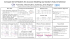### Blower Package Pressure and Vacuum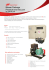### SES-300-XXX-ATEX eng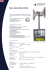### Harvard Graduates to Intelligent AV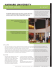### Christmas Sale - best in music education center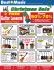### KX2500 - KohlerPower.com